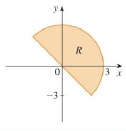Chapter 15.3, Problem 4E

Chapter
Section
Textbook Problem

A region R is shown. Decide whether to use polar coordinates or rectangular coordinates and write ∬ R f ( x ,   y )   d A as an iterated integral, where f is an arbitrary continuous function on R.4.To determine

To write: An iterated integral by deciding whether to use polar coordinates or rectangular coordinates.

Explanation

Formula used:

If f is a polar rectangle R given by 0arb,αθβ, where 0βα2π , then, Rf(x,y)dA=αβabf(rcosθ,rsinθ)rdrdθ (1)

Calculation:

Since the given region is the semicircle, it is easier to use polar coordinates.

From the figure given in the problem, it is observed that radius r ranges from 0 to 3 and θ varies from π4 to 3π4 .

Therefore, the polar coordinates is, R={(r,θ)|0r3,π4θ3π4}

Still sussing out bartleby?

Check out a sample textbook solution.

See a sample solution

The Solution to Your Study Problems

Bartleby provides explanations to thousands of textbook problems written by our experts, many with advanced degrees!

Get Started

In Exercises 1728, use the logarithm identities to obtain the missing quantity.

Finite Mathematics and Applied Calculus (MindTap Course List)

If f is continuous on [a, b] show that 2abf(x)f(x)dx=[f(b)]2[f(a)]2

Single Variable Calculus: Early Transcendentals, Volume I

In Exercises 63-68, use the graph of the function f to determine limxf(x) and limxf(x) 64.

Applied Calculus for the Managerial, Life, and Social Sciences: A Brief Approach

∫(x − cos x)dx = 1 − sin x + C 1 + sin x + C

Study Guide for Stewart's Single Variable Calculus: Early Transcendentals, 8th

For and

Study Guide for Stewart's Multivariable Calculus, 8th